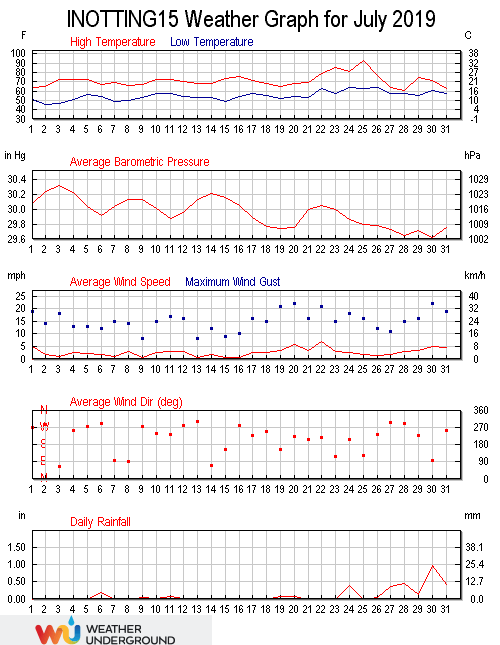July 2019 was warmer than average (17.3°C versus an average of 16.4), wetter than average (82.80 mm versus an average total of 44.78) and calmer than average (a wind run of 1944 miles versus an average of 2044).

The record temperature for this location was smashed in July 2019 when the thermometer reached 34.1°C at 18:37 on the 25th. This easily beat the previous record of 32.7°C set on July 1st 2015. This is only the fifth time that a temperature in excess of 30°C has been recorded here. The minimum temperature was 7.9°C recorded at 05:00 on the 2nd. The average temperature in the month was 17.3°C, 0.8°C above the July mean. The average daily high was 21.8°C, 0.5°C above the mean, with the average daily low being 12.9°C, 0.9°C above the mean. The warmest day was the 25th with an average of 26.4°C, with the coolest day being the 2nd with an average of 13.6°C.

The average wind chill in the month was 17.3°C and the average dew point was 13.5°C. The high dew point was 22.3°C recorded at 18:29 on the 25th, with the low dew point being 6.3°C at 05:11 on the 3rd. There were 218.2 hours of sunshine in the month, with the sunniest day being the 3rd when 11.58 hours was recorded. The maximum heat index was a record 38.5°C recorded at 19:29 on the 25th. this beat the previous high of 35.3°C set in 2015.

Rainfall in July was 82.8 millimetres, well above the July average of 46.6 millimetres, recorded on 15 days. The wettest day was the 30th when 25.0 millimetres of rain was recorded, making it the wettest day since 26.4 millimetres fell on August 8th 2017. The year to date total is now 358.2 millimetres, 117% of the average for the end of July. The highest rain rate was 123.8 millimetres per hour at 21:11 on the 30th.

The highest barometric pressure of 1027.3 millibars was at 11:27 on the 3rd. The lowest pressure was 999.3 millibars at 19:47 on the 30th. The average pressure in the month was 1014.2 millibars. The highest humidity was 96% at 16:21 on the 19th, and the monthly average humidity was 80%. The lowest humidity was 43% at 15:23 on the 25th.

Winds were lower than average in the month, with the highest wind gust speed being 24 mph recorded at 14:51 on the 1st, 1.8 mph below the month average. The maximum wind speed was 11 mph at 12:21 on the 30th, 4.1 mph below the mean. The wind run was 1943.9 miles, well below the July average of 2319.1 miles. The average gust speed in the month was 4.2 mph, 1.2 mph below the mean. The average wind speed was 2.7 mph, 0.5 mph below the mean. The predominant direction was south south-westerly (210°).

The maximum high solar radiation recorded at 12:58 on the 7th was 1183 w/m². The average solar radiation for the month was 177 w/m². The highest U.V. Index was 6.8 recorded at 12:42 on the 2nd, the highest since 7.0 was recorded on August 3rd 2018.This site uses Akismet to reduce spam. Learn how your comment data is processed.